Related Articles

# Weiler Atherton – Polygon Clipping Algorithm

• Last Updated : 26 Aug, 2019

### Background:

Weiler Atherton Polygon Clipping Algorithm is an algorithm made to allow clipping of even concave algorithms to be possible. Unlike Sutherland – Hodgman polygon clipping algorithm, this algorithm is able to clip concave polygons without leaving any residue behind.

### Algorithm:

``` 1. First make a list of all intersection points namely i1, i2, i3, ...
2. Classify those intersection points as entering or exiting.
3. Now, make two lists, one for the clipping polygon, and the other
for the clipped polygon.
4. Fill both the lists up in such a way that the intersection points
lie between the correct vertices of each of the polygon. That is
the clipping polygon list is filled up with all the vertices of
the clipping polygon along with the intersecting points lying
between the corresponding vertices.
5. Now, start at the 'to be clipped' polygon's list.
6. Choose the first intersection point which has been labelled as
an entering point. Follow the points in the list (looping back to
the top of the list, in case the list ends) and keep on pushing
them into a vector or something similar of the sorts. Keep on following
the list until an exiting intersection point is found.
7. Now switch the list to the 'polygon that is clipping' list, and find
the exiting the intersection that was previously encountered. Now keep
on following the points in this list (similar to how we followed the
previous list) until the entering intersection point is found (the
one that was found in the previous 'to be clipped' polygon's list).
8. This vector now formed by pushing all the encountered points in the
two lists, is now the clipped polygon (one of the many clipped
polygons if any of the clipping polygons is concave).
9. Repeat this clipping procedure (i.e. from step 5) until all the
entering intersection points have been visited once.
```

### Explanation:

1. Finding all the intersection points and grouping them
Here, let there be a polygon ABCD and another polygon VWXYZ. Let ABCD be the clipping polygon and let VWXYZ be the clipped polygon.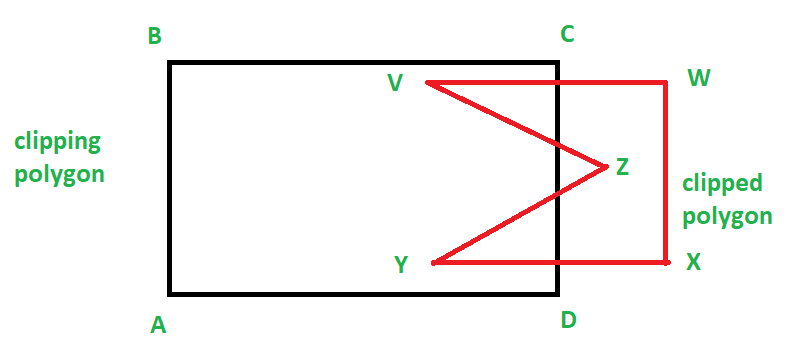So, we can find the intersection points using any method. For example, we can find the intersecting points separately and then find for each intersecting point find if it is entering or leaving, or, we can use Cyrus Beck and find all the intersecting points and also get if a point is entering or exiting. Refer Cyrus Beck for more information on this algorithm.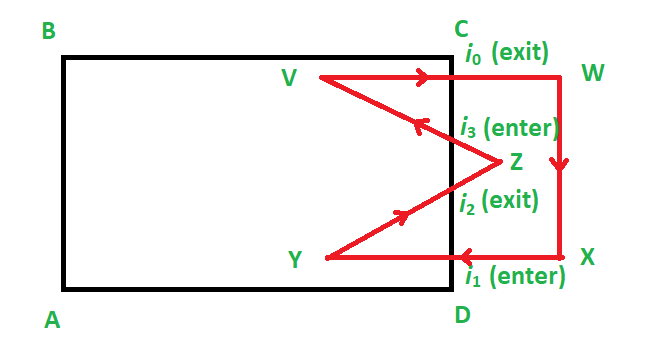2. Making and filling of two lists
Now, we make two lists. One for the clipping polygon and one for the clipped polygon.
Now this is how we fill it: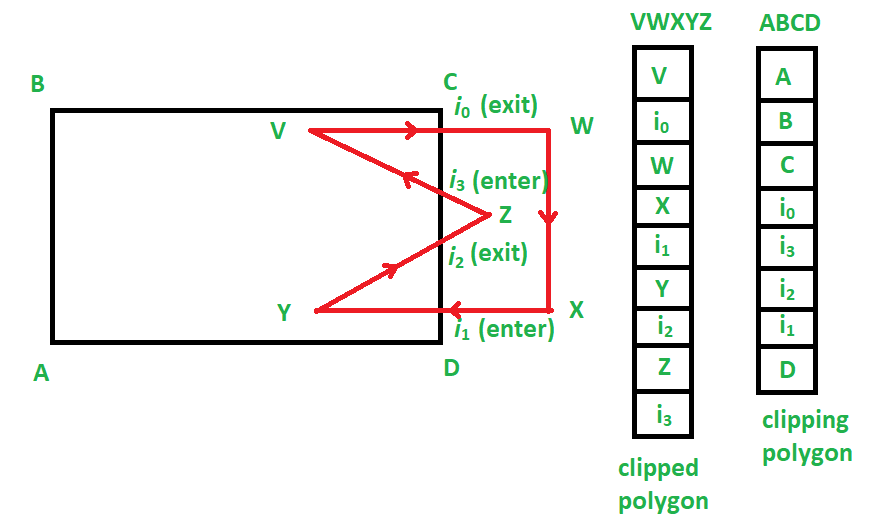3. Running of the algorithm
We start at the clipped polygon’s list, i.e. VWXYZ.
Now, we find the first intersecting point that is entering. Hence we choose i1.
From here we begin the making of the list of vertices (or vector) to make a clipped sub-polygon.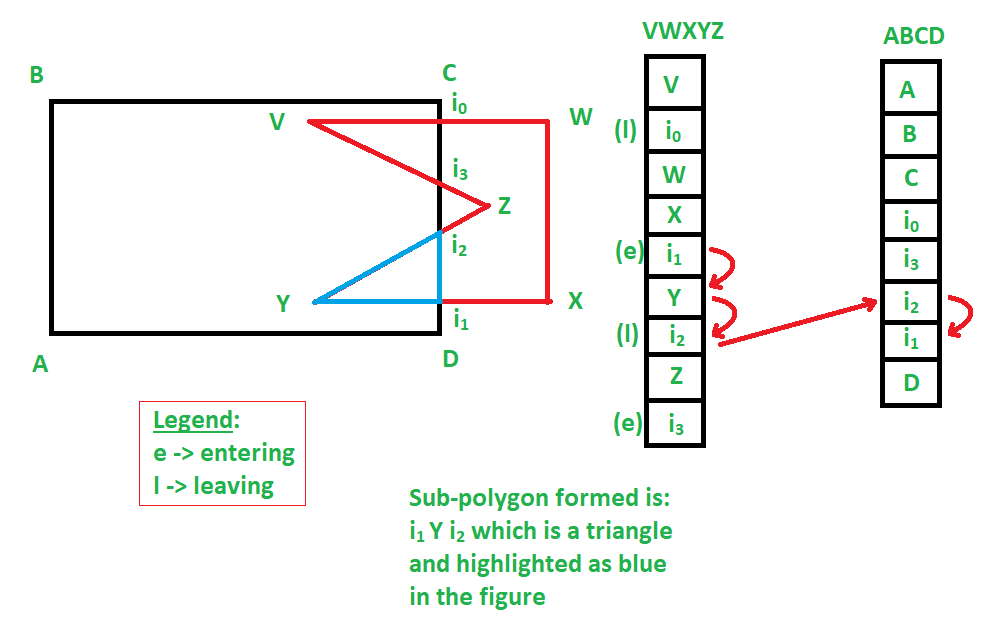According to the given example, i1 Y i2 is a clipped sub-polygon.
Similarly, we get: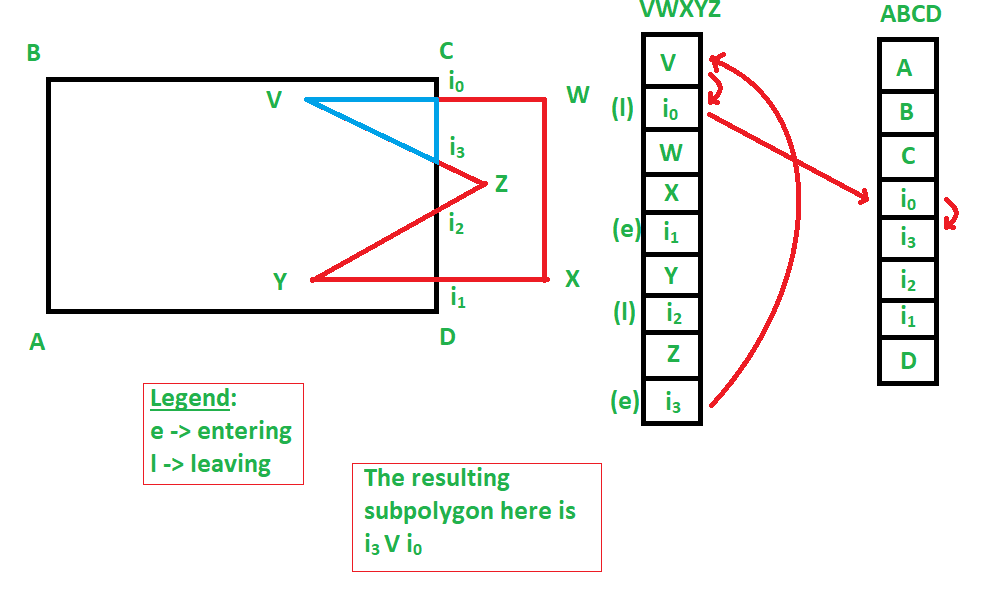i0 V i3 as another sub-polygon also.

Hence, we were able to get two sub-polygons as a result of this polygon clipping, which involved a concave polygon, which resulted in: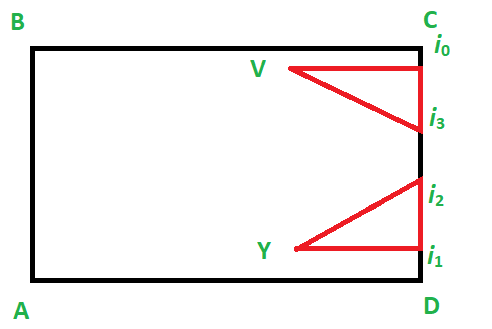Similarly, this clipping works for convex polygons.

### Limitations:

This polygon clipping algorithm does not work for self – intersecting polygons, although some methods have been proposed to be able to solve this issue also, and have successfully worked.

### Example:

Let V1V2V3V4V5V6 be the clipping window and P1 P2 P3 P4 P5 P6 be the polygon.
Now, here is how the algorithm will operate.
Two lists are generated, one for the clipping window, and the other for the polygon. We will start from the polygon’s list. (Note that the polygon’s list only contains the polygon’s vertices and the intersections and similarly for the clipping window’s list.)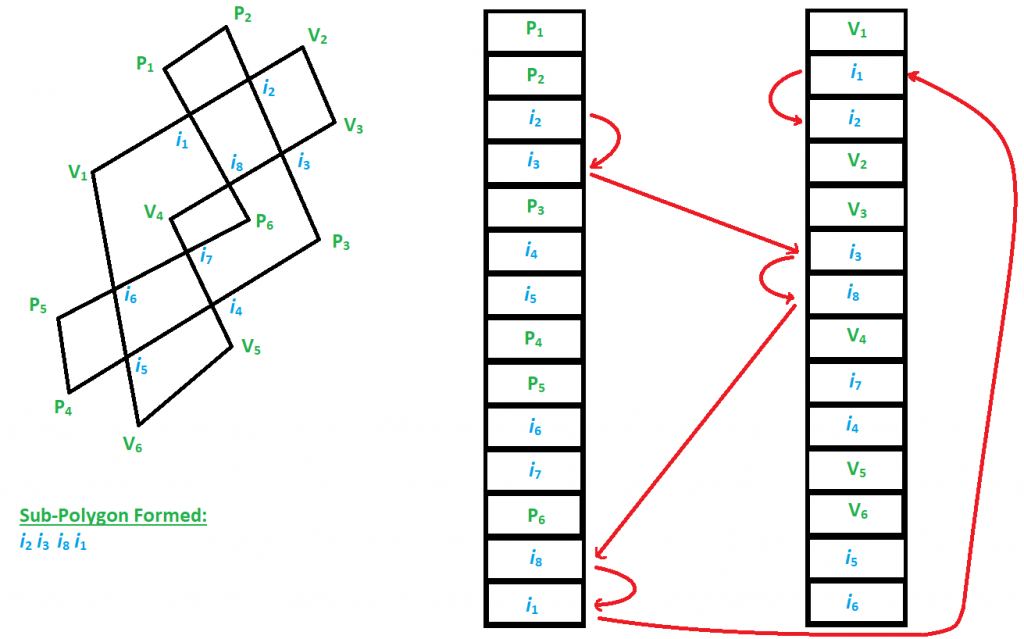So, according to the algorithm, the first polygon to be formed will be i2 i3 i8 i1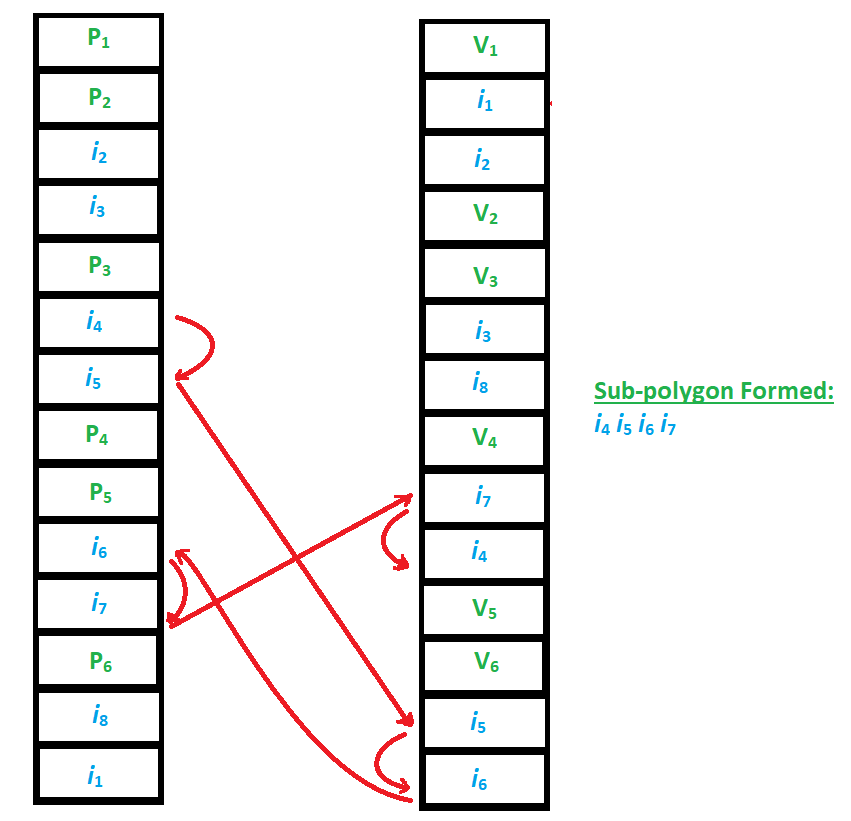Then the next subpolygon, i4 i5 i6 i7.

The output will look like: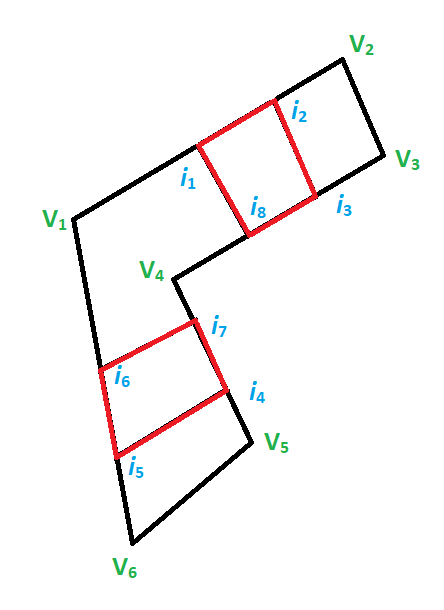### Citation:

K. Weiler and P. Atherton. 1988. Hidden surface removal using polygon area sorting. In Tutorial: computer graphics; image synthesis, Kenneth I. Joy, Charles W. Grant, Nelson L. Max, and Lansing Hatfield (Eds.). Computer Science Press, Inc., New York, NY, USA 209-217.

For implementation of Weiler Atherton, check Implementation

My Personal Notes arrow_drop_up